# A Two-Parameter Power Garima Distribution with Properties and Applications

Berhane Abebe, Mussie Tesfay, Tesfalem Eyob and Rama Shanker*

Department of Statistics, Eritrea

Received Date: January 05, 2019;  Published Date: January 24, 2019

#### Abstract

In this article we propose a two-parameter power Garima distribution which includes Garima distribution as particular case. Its statistical properties including behavior of its probability density function for varying values of parameters, moments, hazard rate function and mean residual life function has been discussed. The estimation of the parameters of the distribution has been discussed using maximum likelihood estimation. Applications of the distribution have been investigated using real lifetime datasets from engineering and medical science.

Keywords:Garima distribution; Moments; Hazard rate function; Mean residual life function; Maximum likelihood estimation; Applications

#### Introduction

Shanker  introduced one parameter lifetime distribution having probability density function (pdf) and cumulative distribution function (cdf) as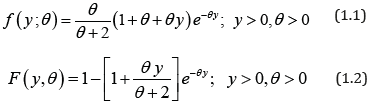Garima distribution (1.1) can be easily expressed as a mixture of exponential (θ ) and gamma (2,θ ) distributions with mixing proportion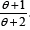. We have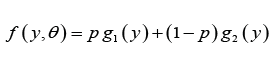whereShanker  has discussed some of its statistical properties including shapes for varying values of parameter, moments, skewness, kurtosis, hazard rate function, mean residual life function, stochastic ordering, mean deviations, order statistics, Bonferroni and Lorenz curves, entropy measure and stressstrength reliability. The condition under which Garima distribution is over-dispersed, equi-dispersed, and under-dispersed has been also discussed along other one parameter lifetime distributions. Maximum likelihood estimation and method of moments have been discussed for estimating its parameter. An application of Garima distribution for modeling life time data from behavioral science has been also discussed in Shanker . Shanker  has also introduced discrete Poisson-Garima distribution and discussed its statistical properties, estimation of parameter and applications. Shanker & Shukla [3,4] have studied size-biased Poisson-Garima distribution and Zero-truncated Poisson-Garima distribution.

The pdf and the cdf of Power Lindley distribution (PLD) obtained by Ghitany et al  are given byIt can be easily shown that at α =1, PLD reduces to one parameter Lindley distribution,introduced by Lindley (1958) having pdf and cdfThe detailed study regarding various statistical properties, estimation of parameter and application of Lindley distribution are available in Ghitany et al. . Shanker et al. (2015) have critical and comparative study on applications of Lindley distribution and exponential distribution to model real lifetimes data from engineering and biomedical sciences. Garima distribution, being one parameter lifetime distribution, has less flexibility to model data of various natures. In the present paper an attempt has been made to obtain two-parameter power Garima distribution using power transformation of Garima distribution which includes one parameter Garima distribution as particular cases. The statistical properties of the distribution including shapes of density for varying values of parameters, the moments, survival function, hazard rate function and mean residual life function have been discussed. The maximum likelihood estimation has been discussed. The goodness of fit of the proposed distribution has been discussed with two real lifetime data set from engineering and medical sciences and fit shows quite satisfactory over other one parameter and two-parameter lifetime distributions.

#### Power Garima Distribution

Assuming the power transformation X = Y1/α in (1.1), the pdf of the random variable X can be obtained asWe would call the density in (2.1) as power Garima distribution (PGD) with parameters θ and α , and it is denoted by PGD(α ,θ ) . Like Garima distribution, PGD is also a convex combination of Weibull(θ ,α ) distribution, a generalized gamma(2,α ,θ ) distribution.

The corresponding cdf of PGD can be obtained as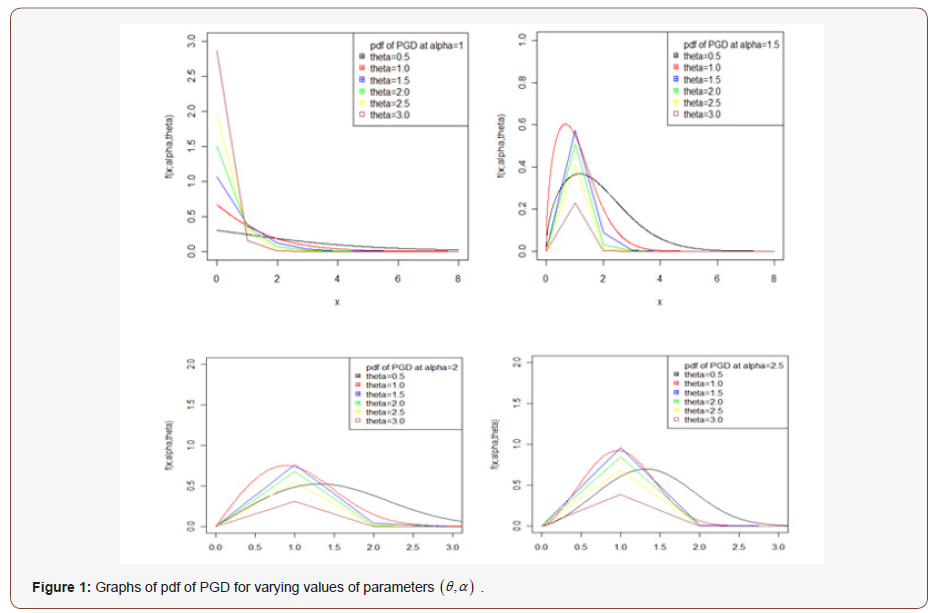The nature of the pdf of PGD has been studied with the help of the graphs for varying values of parameters and presented in Figure 1.

Further, the nature of the cdf of PGD has also been studied with the help of the graphs for varying values of parameters and presented in Figure 2.#### Moments of Power Garima Distribution

Using the mixture representation in (2.2), the rth moment about the origin,of PGD can be obtained asIt should be noted that at α =1, the above expression reduces to the rth moment about origin of Garima distribution given by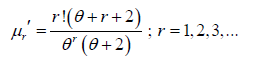Thus the first four moments about origin of the PGD are thus obtained as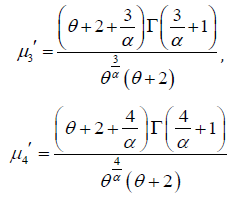Therefore, the variance of PGD can be obtained asThe higher order central moments are not being given here because their expressions are big. However, higher order central moments, if required, can be easily obtained using relationship between moments about mean and moments about origin. Finally, coefficient of variation, skewness, kurtosis and index of dispersion, if needed, can be obtained using their formulae in terms of central moments.

#### Reliability Properties of Power Garima Distribution

The survival function of PGD can be obtained as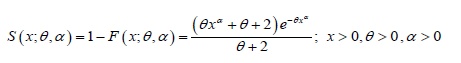The hazard rate function h(x;θ ,α ) and the mean residual function

m(x;θ ,α ) of PGD are given respectively as:It can be easily verified that at x = 0 it reduces to the expression for the mean of PGD. The nature of hazard rate function of PGD for varying values of parameters has been shown graphically in figure 3. The graphs of hazard rate function of PGD are monotonically increasing for varying values of parameters. Again, the nature of mean residual life function of PGD for varying values of parameters has been shown in figure 4. The graphs of mean residual life function of PGD are monotonically decreasing for varying values of parameters.#### Maximum Likelihood Estimation of Parameters of Power Garima Distribution

Suppose ( x1, x2,.....xn> ) be a random sample of size n From PGD (θ ,α ) The log-likelihood function of PGD can be expressed as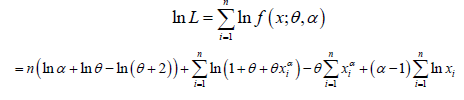The maximum likelihood estimatesof parameters (α ,θ ) of PGD are the solution of the following log-likelihood equations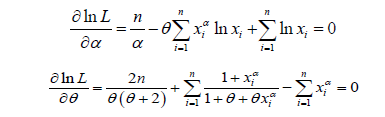These two natural log likelihood equations do not seem to be solved directly, because they cannot be expressed in closed forms. The (MLE’s)of (θ ,α ) can be computed directly by solving the natural log likelihood equation using Newton-Raphson iteration using R-software till sufficiently close values of θˆ andαˆ are obtained.

#### Goodness of Fit of Power Garima Distribution

In this section, the goodness of fit of PGD using maximum likelihood estimates of parameters has been discussed with two real datasets. The goodness of fit has been compared with other one parameter and two-parameter lifetime distributions.

##### Data Set 1

The data set represents the strength of 1.5cm glass fibers measured at the National Physical Laboratory, England. Unfortunately, the units of measurements are not given in the paper, and they are taken from Smith & NaylorIn order to compare the considered distributions, values of MLEalong with their standard errors, -2ln L, AIC (Akaike Information Criterion), K-S (Kolmogorov-Smirnov Statistics) and p-values for the real lifetime dataset 1 have been computed and presented in Table1. The best distribution is the distribution corresponding to lower values of -2ln L, AIC, K-S statistic (Table 1).

Table 1: MLE’s, -2ln L, se, AIC, K-S statistic, and P-value of the fitted distribution of dataset1.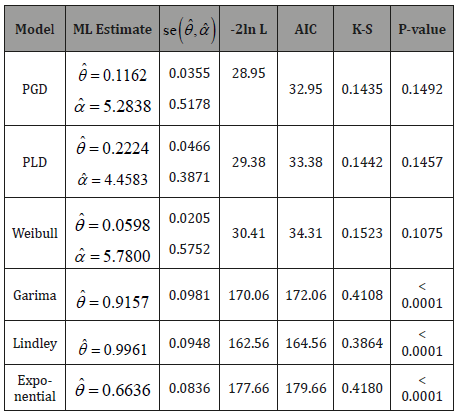The Variance-Covariance matrix and 95% confidence interval (CI’s) for the parametersand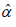of PGD for the given dataset 1 has been presented in Table 2.

Table 2: Variance-Covariance matrix and 95% confidence interval (CI’s) for the parametersandof PGD.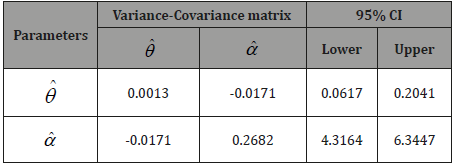In order to see the closeness of the fit given by one parameter exponential, Lindley and Rama distributions and two-parameter Weibull distribution, PLD and PGD, the fitted plot of pdfs of these distributions for the given dataset have been shown in Figure 5. It is also obvious from the fitted plots of the distributions along with the histogram of the original dataset that PGD gives much closer fit as compared to other considered distributions (Figure 5).

##### Data Set 2

The following dataset represents waiting time (in minutes) of 65 dental patients, waiting before OPD (Out Patient Diagnosis) from the 25th to 29th December, 2017 at Halibet Hospital, Asmara, available in the master thesis of Berhane Abebe , Department of Statistics, College of Science, Eritrea Institute of Technology, Eritrea .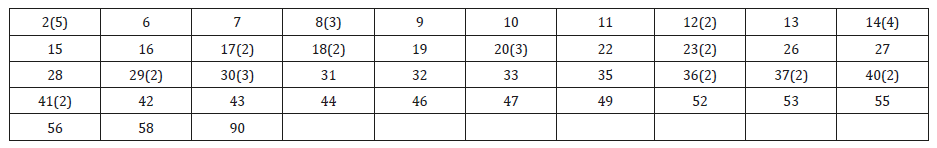The maximum likelihood estimates of parameters, standard error of estimates of parameters, AIC, K-S and p-values of the fitted distributions for dataset 2 are shown in Table 3.

Table 3: MLE’s, -2ln L, se, AIC, K-S statistic, and P-value of the fitted distribution of dataset2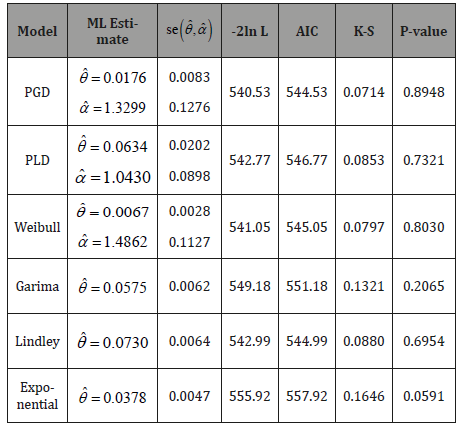The Variance-Covariance matrix and 95% confidence interval (CI’s) for the parametersandof PGD for the given dataset 2 has been presented in Table 4.

Table 4:Variance-Covariance matrix and 95% confidence interval (CI’s) for the parametersandof PGD.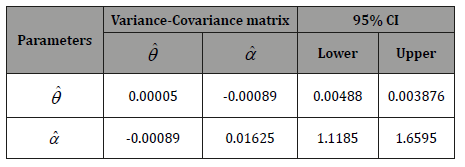In order to see the closeness of the fit given by one parameter exponential, Lindley and Garima distributions and two-parameter Weibull distribution, introduced by Weibull (1951), PLD and PGD, the fitted plot of pdfs of these distributions for the given dataset have been shown in Figure 6. It is also obvious from the fitted plots of the distributions along with the histogram of the original dataset that PGD gives much closer fit as compared to other considered distributions (Figure 6).#### Conclusion

A two-parameter continuous Power Garima distribution (PGD) has been introduced which includes Garima distribution, introduced by Shanker , as a particular case. The statistical and reliability properties including shapes of density for varying values of parameters, the moments about the origin, the variance, survival function, hazard rate function, mean residual function of PGD have been discussed. The method of maximum likelihood for estimating the parameters has been discussed. Finally, the goodness of fit of PGD has been discussed with two real lifetime datasets and the fit has been found quite satisfactory as compared to one parameter Garima, Lindley and Exponential distributions and two-parameter Power Lindley distribution (PLD) and Weibull distribution. Therefore, PGD can be considered as an important lifetime distribution for modeling lifetime data from engineering and biomedical sciences.

None.

#### Conflict of Interest

No conflict of interest.

Article Details
Citation
Keywords
Scroll to
Scroll to Top